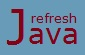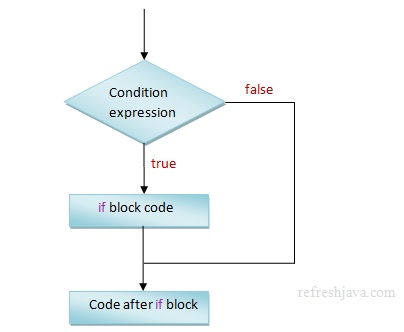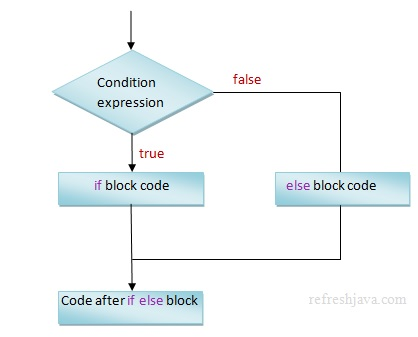# Java selection or control flow statements(if and if-else)

Sometime as a programmer you want to execute one or more lines of code on basis of certain condition. For an example, you may want that if a number `num1` is greater than `0`, print a message in console as "num1 is a positive number" by executing code `System.out.println("num1 is a positive number");` To accomplish such scenarios, java provide a keyword known as `if` keyword which allows programmers to execute a set of statements on certain condition. The `if` statement asks the computer to execute the instructions given inside it's block only when the condition is `true`. The syntax of `if` keyword is:

``` `if`(condition) {
`// java code to run inside if block`
}```

Here condition is a boolean expression which returns either `true` or `false`. If it returns `true`, the code written inside `if` block get's executed and if it returns `false`, `if` block code will not be executed. The boolean expressions could be like `a>0`, `(a+b)>10`, `(a-b)>0` etc. These expressions returns either `true` or `false`.

### What is if block in java ?

Everything that comes inside matching curly brackets { } after `if` keyword is the part of `if` statement which is also called as `if` block in java.

### Flowchart of if statement in Java### if Statement program in java

```` class` IfStatement {
`public static void` main(String [] args) {
`int` num1 = 20;
`if`(num1 > 0) {
System.out.println(`"num1 is a positive number"`);
System.out.println(`"Value of num1 = "`+num1);
}
`if`(num1 < 0) {
System.out.println(`"num1 is a negative number"`);
System.out.println(`"Value of num1 = "`+num1);
}
System.out.println(`"statement after if block"`);
}
}```

Output :

num1 is a positive number
Value of num1 = 20
statement after if block

### Can I write multiple conditions in if statement in java ?

Yes, We can use multiple conditions in `if` statement by using logical AND(&&) and logical OR(||) operator. For example `if`(a > 10 && a < 20) is a valid boolean condition. Refer the program given below.

### Can we use if statement without curly braces ?

Yes, we can if we have only a single line of code for `if` statement. In other words if we don't use the curly braces after `if` statement, the `if` condition will be applied only on the first line of code after `if` statement. Refer the program given below.

### What happens if we put semicolon after if statement in Java ?

Though it will compile successfully but the `if` statement will end up there itself. It simply means the `if` condition won't be applied on the statements of `if` block, so these statements will be executed always. Refer the program given below :

```` class` IfStatementUsage {
`public static void` main(String [] args) {
`int` a = 15, b = 10;
`if`(a > 0)  `// no curly brances`
System.out.println(`"a > 0"`); `// if condition is applied on this line only`
System.out.println(`"a = "`+a);

`if`(a > 10 && a < 20)
System.out.println(`"a > 10 and < 20"`);
`if`(a > 0 || b > 0)
System.out.println(`"a > 0 or b > 0"`);
`if`(a > 0 && a < 20 && a > b)
System.out.println(`"a > 0 and < 20 and a > b"`);
`if`(a < 0); {  `// semicolon ends the if statement`
System.out.println(`"Printed even the if condition is false"`);
}
}
}```

Output :

a > 0
a = 15
a > 10 and < 20
a > 0 or b > 0
a > 0 and < 20 and a > b
Printed even the if condition is false

## if-else (if-then-else) condition in Java

Sometime as a programmer you want to execute a set of statements when a condition is `true` and if that is not `true` then you want to execute some other set of statements. To accomplish this java provides `if` then `else` statement. The `else` block get's executed only when the condition in `if` statement is `false`. Programmer can also read this as "`if` something is `true` execute this `else` execute that". The syntax of `if`-then-`else` statement is:

``` `if`(condition) {
`// java code to run inside if block`
}
` else` {
`// java code to run inside else block`
}    ```

### Can we use else without if in java ?

No, you can not use `else` statement without `if` statement. The `else` statement must be used along with `if` statement.

### if else flowchart in Java### if else program in Java

```` class` IfElseStatement {
`public static void` main(String [] args) {
`int` num1 = 20, num2 = 15;
`if`(num1 == num2) {
System.out.println(`"num1 is equal to num2"`);
}
`else` {
System.out.println(`"num1 is not equal to num2"`);
}
System.out.println(`"code after if else block"`);
}
}```

Output:

num1 is not equal to num2
code after if else block

### Do you need else after if in java ?

Not necessarily, it depends on program requirement whether you want to use `else` after `if` or not, otherwise `if` statement can be used alone.

### Can we use if statement with numbers like if(10), if(20.5) etc ?

No, you can not use numbers in `if` condition because java compiler expects only boolean value in `if` condition.

★★★
• Java if else statements are also known as decision making or conditional statements.
• Ensure that all letters of conditional statements are small. For example If, Else, IF etc are not valid java keywords.